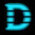## Monday, December 14, 2015

### Python 3 check using type([1.0 for i in ]) is type([1 for i in ])

Yesterday I've stumbled on a very interesting tweet: "guess why: (by arigo) so a way to know "are we on python 3" is: type([1.0 for i in ]) is type([1 for i in ])". Original link is https://twitter.com/fijall/status/675651525000175616

In Python 3 the result is:
```>>> type([1.0 for i in ]) is type([1 for i in ])
>>> True
```
but in Python 2:
```>>> type([1.0 for i in ]) is type([1 for i in ])
>>> False
```
It is interesting that items' types in Python 3 are both float:
```>>> type([1.0 for i in ]), type([1 for i in ])
>>> (<class 'float'>, <class 'float'>)
```
but in Python 2 they are different:
```>>> type([1.0 for i in ]), type([1 for i in ])
>>> (<type 'float'>, <type 'int'>)
```
The first part of a secret is that in Python 3 list comprehensions have their own scope, but in Python 2 they haven't. Guido van Rossum wrote about this "dirty little secret" here.
In Python 3 for list comprehensions a special code object listcomp was created.

And the second part of a secret is that both listcomp code objects from this example are located at the same address, because declared on the one line in code.
Here is a bytecode (I've used dis module to get is):
```...
6 LOAD_CONST       1 (<code object <listcomp> at 0x7fd91d983270, file "main.py", line 3>)
...
35 LOAD_CONST       1 (<code object <listcomp> at 0x7fd91d983270, file "main.py", line 3>)
...
```
List comprehensions [1.0 for i in ] and [1 for i in ] are on one line have the same address (address of the first listcomp code object), because their listcomp code objects are the same.
Update: As Rhomboid correctly noticed in the discussion on reddit, constants for current code object are stored in dictionary (although displayed as tuple) and the same code object constants are folded.
As hash values for [1.0 for i in ] and [1 for i in ] are the same, when addition to the dict is performed - items' values are compared using code_richcompare methods (in this way python dict resolves collisions).

Here is how hash for code object is calculated (from codeobject.c):
```static Py_hash_t
code_hash(PyCodeObject *co)
{
Py_hash_t h, h0, h1, h2, h3, h4, h5, h6;
h0 = PyObject_Hash(co->co_name);
if (h0 == -1) return -1;
h1 = PyObject_Hash(co->co_code);
if (h1 == -1) return -1;
h2 = PyObject_Hash(co->co_consts);
if (h2 == -1) return -1;
h3 = PyObject_Hash(co->co_names);
if (h3 == -1) return -1;
h4 = PyObject_Hash(co->co_varnames);
if (h4 == -1) return -1;
h5 = PyObject_Hash(co->co_freevars);
if (h5 == -1) return -1;
h6 = PyObject_Hash(co->co_cellvars);
if (h6 == -1) return -1;
h = h0 ^ h1 ^ h2 ^ h3 ^ h4 ^ h5 ^ h6 ^
co->co_argcount ^ co->co_kwonlyargcount ^
co->co_nlocals ^ co->co_flags;
if (h == -1) h = -2;
return h;
}
```
Here is how code objects are compared (from codeobject.c):
```static PyObject *
code_richcompare(PyObject *self, PyObject *other, int op)
{
PyCodeObject *co, *cp;
int eq;
PyObject *res;

if ((op != Py_EQ && op != Py_NE) ||
!PyCode_Check(self) ||
!PyCode_Check(other)) {
Py_RETURN_NOTIMPLEMENTED;
}

co = (PyCodeObject *)self;
cp = (PyCodeObject *)other;

eq = PyObject_RichCompareBool(co->co_name, cp->co_name, Py_EQ);
if (eq <= 0) goto unequal;
eq = co->co_argcount == cp->co_argcount;
if (!eq) goto unequal;
eq = co->co_kwonlyargcount == cp->co_kwonlyargcount;
if (!eq) goto unequal;
eq = co->co_nlocals == cp->co_nlocals;
if (!eq) goto unequal;
eq = co->co_flags == cp->co_flags;
if (!eq) goto unequal;
eq = co->co_firstlineno == cp->co_firstlineno;
if (!eq) goto unequal;
eq = PyObject_RichCompareBool(co->co_code, cp->co_code, Py_EQ);
if (eq <= 0) goto unequal;
eq = PyObject_RichCompareBool(co->co_consts, cp->co_consts, Py_EQ);
if (eq <= 0) goto unequal;
eq = PyObject_RichCompareBool(co->co_names, cp->co_names, Py_EQ);
if (eq <= 0) goto unequal;
eq = PyObject_RichCompareBool(co->co_varnames, cp->co_varnames, Py_EQ);
if (eq <= 0) goto unequal;
eq = PyObject_RichCompareBool(co->co_freevars, cp->co_freevars, Py_EQ);
if (eq <= 0) goto unequal;
eq = PyObject_RichCompareBool(co->co_cellvars, cp->co_cellvars, Py_EQ);
if (eq <= 0) goto unequal;

if (op == Py_EQ)
res = Py_True;
else
res = Py_False;
goto done;

unequal:
if (eq < 0)
return NULL;
if (op == Py_NE)
res = Py_True;
else
res = Py_False;

done:
Py_INCREF(res);
return res;
}
```
For the first list comprehension code object variables are the next:
```co_name = <listcomp>
co_argcount = 1
co_kwonlyargcount = 0
co_nlocals = 2
co_flags = 83
co_firstlineno = 3
co_code = b'g\x00\x00|\x00\x00]\x0c\x00}\x01\x00d\x00\x00\x91\x02\x00q\x06\x00S'
co_consts = (1.0,)
co_names = ()
co_varnames = ('.0', 'i')
co_freevars = ()
co_cellvars = ()
```
And for the second one code object variables are the same, except co_consts:
`co_consts = (1,)`
But as 1.0 == 1, and tuples (1.0,) and (1,) are equal. Therefore Python considers the second code object as duplicate of the first one, and its address is the same. So identity operator "is" returns True for the same objects.

And that is why in Python 3 the next expressions will also be valid.
```>>> a = type([1.0 for i in ]); b = type([1 for i in ])
>>> print(a, b)
>>> <class 'float'> <class 'float'>

>>> type([1 for i in ]) is type([True for i in ])
>>> True```
See also: how hash values are calculated in Python read in my article Python hash calculation algorithms

1.Python3 is inconsistent even within this inconsistency. No implicit coercion for imaginary literals:

>>> type([1.0 for i in ]); type([1+0j for i in ])

>>> 1.0 == 1+0j
True

1.In your example types are float and complex, because co_code for list comprehension with float/int/bool value and for list comprehension with complex value are different.
For float/int/bool: b'g\x00\x00|\x00\x00]\x0c\x00}\x01\x00d\x00\x00\x91\x02\x00q\x06\x00S'
But for complex: b'g\x00\x00|\x00\x00]\x0c\x00}\x01\x00d\x02\x00\x91\x02\x00q\x06\x00S'
Therefore objects are not equal, although the values are:
>>> 1.0 == True == 0j+1 == 1
>>> True